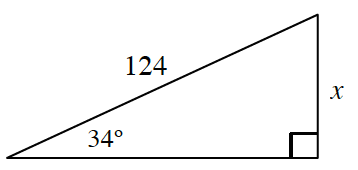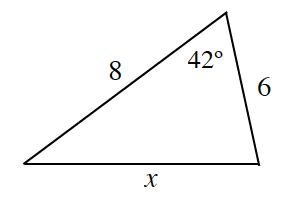### Home > INT3 > Chapter 10 > Lesson 10.2.1 > Problem10-102

10-102.

Solve for $x$ in each diagram. Homework Help ✎

1.Refer to problem 10-57 for help on the use of trigonometric functions.

1.Use the Law of Cosines to solve for $x$.

$x ≈ 5.35$